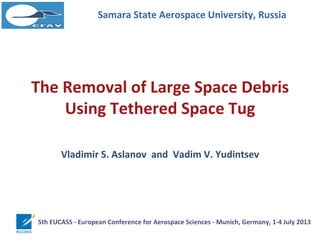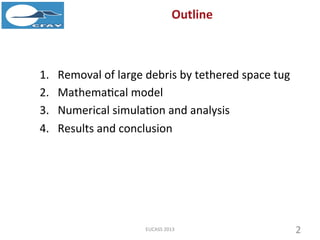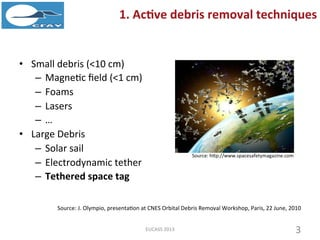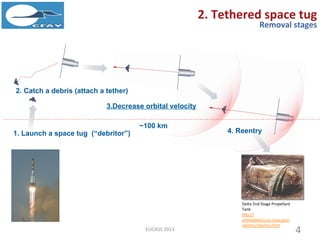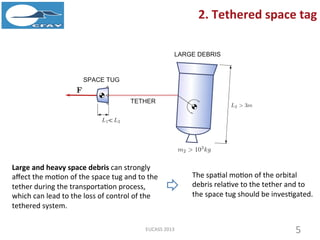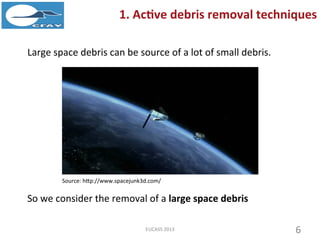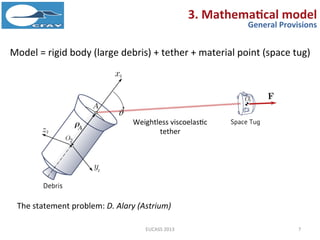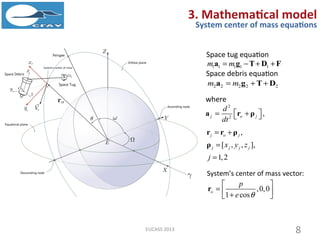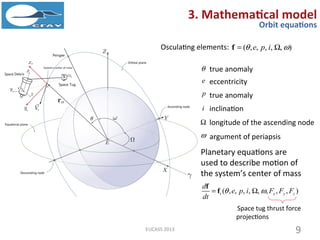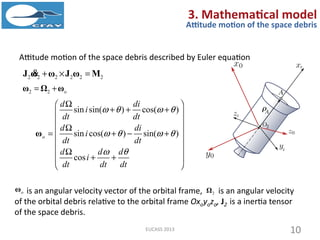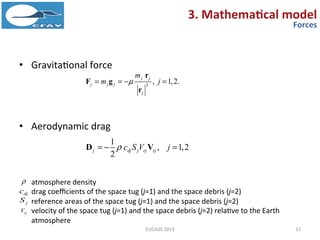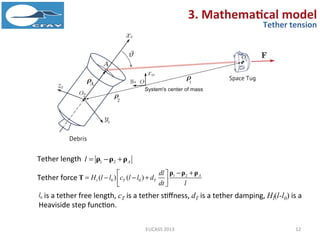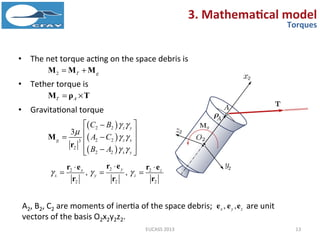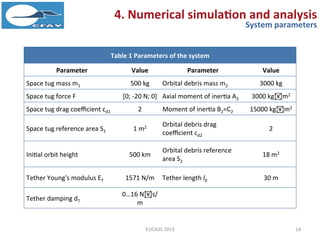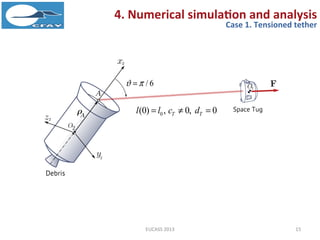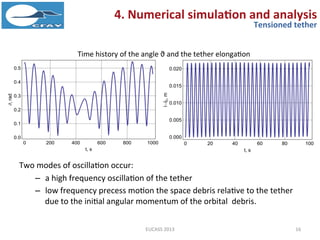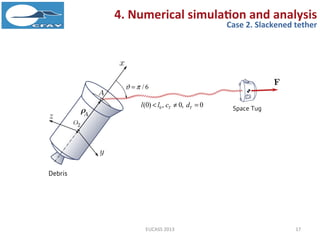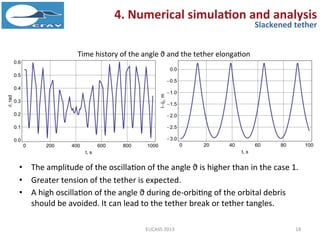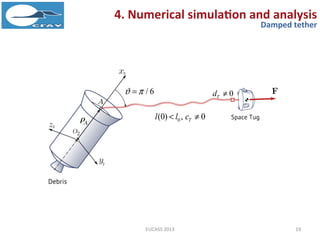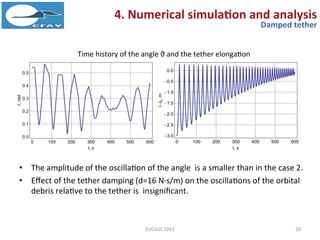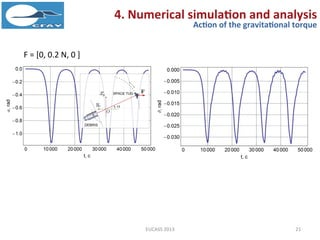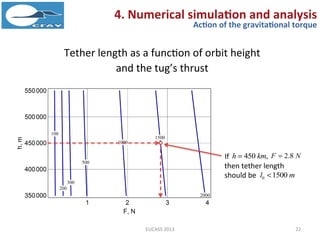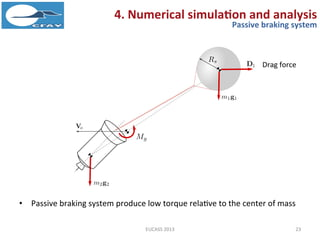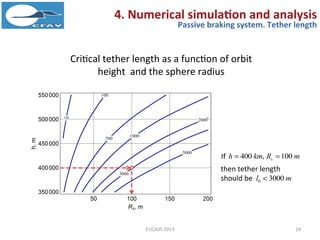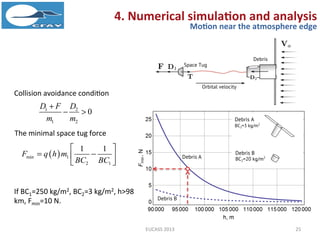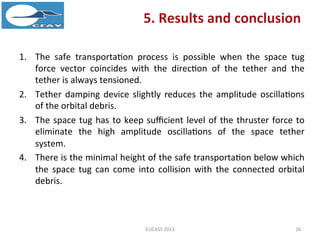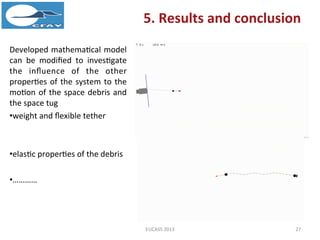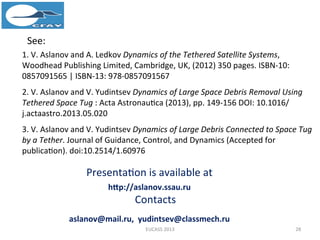1 of 28

### The Removal of Large Space Debris Using Tethered Space Tug

1. The Removal of Large Space Debris Using Tethered Space Tug Samara State Aerospace University, Russia Vladimir S. Aslanov and Vadim V. Yudintsev 5th Eucass - European Conference for Aerospace Sciences - Munich, Germany, 1-4 July 2013
2. 1. Active debris removal techniques 2. Tethered space tug 3. Mathematical model 4. Numerical simulation and analysis 5. Results and conclusion 2 Outline
3. • Small debris (<10 cm) – Magnetic field (<1 cm) – Foams – Lasers – … • Large Debris – Solar sail – Electrodynamic tether – Tethered space tag Source: J. Olympio, presentation at CNES Orbital Debris Removal Workshop, Paris, 22 June, 2010 Source: http://www.spacesafetymagazine.com 3 1. Active debris removal techniques
4. Large space debris body can be source of a lot of small debris. Source: http://www.spacejunk3d.com/ 4 1. Active debris removal techniques So we consider the removal of a large space debris
5. 1. Launch a space tug (“debritor”) 2. Catch a debris (attach a tether) 3.Decrease orbital velocity 4. Reentry ~100 km 5 2. Tethered space tug Removal stages Delta 2nd Stage Propellant Tank http://orbitaldebris.jsc.nasa.go v/reentry/reentry.html
6. 6 2. Tethered space tag Large and heavy space debris can strongly affect the motion of the space tug and to the tether during the transportation process, which can lead to the loss of control of the tethered system. The spatial motion of the orbital debris relative to the tether and to the space tug should be investigated.
7. 7 3. Mathematical model General Provisions  Model = rigid body (large debris) + tether + material point (space tug) Weightless viscoelastic tether
8. 1 1 1 1 1m m   a g T D F 2 2 2 2 2m m  a g T D 2 2 ,j o j d dt    a r ρ , [ , , ], 1,2 j o j j j j jx y z j     r r ρ ρ ,0,0 1 cos o p e        r 3. Mathematical model System center of mass equations where 8 Space tug equation Space debris equation System’s center of mass vector:
9. ( , , , , , )e p i  f Orbital elements 9 3. Mathematical model Orbit equations Planetary equations are used to describe motion of the system’s center of mass Space tug thrust force projections ( , , , , , , , , )t x y z d e p i F F F dt    f f  true anomaly e eccentricity p true anomaly i inclination  longitude of the ascending node  argument of periapsis
10. Attitude motion of the space debris described by Euler equation 2 2 2 2 2 2  J ω ω J ω M 2 2 o ω Ω ω sin sin( ) cos( ) sin cos( ) sin( ) cos o d di i dt dt d di i dt dt d d d i dt dt dt                                        ω is an angular velocity vector of the orbital frame, is an angular velocity of the orbital debris relative to the orbital frame Oxoyozo, J2 is a inertia tensor of the space debris. oω 2Ω 10 3. Mathematical model Attitude motion of the space debris
11. • Gravitational force • Aerodynamic drag 3 , 1,2. j j j j j j m m j    r F g r 11 1 , 1,2 2 j dj j rj rjc S V j  D V 3. Mathematical model Forces  atmosphere density drag coefficients of the space tug (j=1) and the space debris (j=2) reference areas of the space tug (j=1) and the space debris (j=2) velocity of the space tug (j=1) and the space debris (j=2) relative to the Earth atmosphere djc jS rjV
12. 1 2 Al   ρ ρ ρTether length 1 2 0 0( ) ( ) A l T T dl H l l c l l d dt l           ρ ρ ρ TTether force 12 3. Mathematical model Tether tension 0l is a tether free length, cT is a tether stiffness, dT is a tether damping, Hl(l-l0) is a Heaviside step function.
13. • The net torque acting on the space debris is • Tether torque is • Gravitational torque       2 2 2 23 2 2 2 3 z y z x x y g C B A C B A                  r M 22 2 2 2 2 , , yx z x y z        r er e r e r r r 2 T g M M M T A M ρ T 13 3. Mathematical model Torques A2, B2, C2 are moments of inertia of the space debris; are unit vectors of the basis O2x2y2z2. , ,x y ze e e
14. Table 1 Parameters of the system Parameter Value Parameter Value Space tug mass m1 500 kg Orbital debris mass m2 3000 kg Space tug force F [0; -20 N; 0] Axial moment of inertia A2 3000 kgm2 Space tug drag coefficient cd1 2 Moment of inertia B2=C2 15000 kgm2 Space tug reference area S1 1 m2 Orbital debris drag coefficient cd2 2 Initial orbit height 500 km Orbital debris reference area S2 18 m2 Tether Young's modulus ET 1571 N/m Tether length l0 30 m Tether damping dT 0…16 Ns/m 14 4. Numerical simulation and analysis System parameters
15. 15 4. Numerical simulation and analysis Case 1. Tensioned tether 0(0) , 0, 0T Tl l c d   / 6 
16. Two modes of oscillation occur: – a high frequency oscillation of the tether – low frequency precess motion the space debris relative to the tether due to the initial angular momentum of the orbital debris. 16 4. Numerical simulation and analysis Tensioned tether Time history of the angle ϑ and the tether elongation
17. 17 4. Numerical simulation and analysis Case 2. Slackened tether 0(0) , 0, 0T Tl l c d   / 6 
18. • The amplitude of the oscillation of the angle ϑ is higher than in the case 1. • Greater tension of the tether is expected. • A high oscillation of the angle ϑ during de-orbiting of the orbital debris should be avoided. It can lead to the tether break or tether tangles. 18 4. Numerical simulation and analysis Slackened tether Time history of the angle ϑ and the tether elongation
19. 19 4. Numerical simulation and analysis Damped tether 0(0) , 0Tl l c  / 6  0Td 
20. • The amplitude of the oscillation of the angle is a smaller than in the case 2. • Effect of the tether damping (d=16 N·s/m) on the oscillations of the orbital debris relative to the tether is insignificant. 20 4. Numerical simulation and analysis Damped tether Time history of the angle ϑ and the tether elongation
21. • If tug’s thrust is low high amplitude oscillation of the tether can occur relative to the r0 direction due to the action of the gravitational torque. • The space tug control system should compensate these high oscillations of the angle a by changing the direction of the thruster force vector F. 21 4. Numerical simulation and analysis Action of the gravitational torque F = [0, 0.2 N, 0 ]
22. Tether length as a function of orbit height and the tug’s thrust 2.8F N450 ,h km 0 1500l m If 22 4. Numerical simulation and analysis Action of the gravitational torque then tether length should be
23. • Passive braking system produce low torque relative to the center of mass 23 4. Numerical simulation and analysis Passive braking system Drag force
24. Critical tether length as a function of orbit height and the sphere radius 100sR m400 ,h km 0 3000l m If 24 4. Numerical simulation and analysis Passive braking system. Tether length then tether length should be
25.  min 1 2 1 1 1 F q h m BC BC        The minimal space tug force 25 4. Numerical simulation and analysis Motion near the atmosphere edge 1 2 1 2 0 D F D m m    Collision avoidance condition If BC1=250 kg/m2, BC2=3 kg/m2, h>98 km, Fmin=10 N.
26. 1. The safe transportation process is possible when the space tug force vector coincides with the direction of the tether and the tether is always tensioned. 2. Tether damping device slightly reduces the amplitude oscillations of the orbital debris. 3. The space tug has to keep sufficient level of the thruster force to eliminate the high amplitude oscillations of the space tether system. 4. There is the minimal height of the safe transportation below which the space tug can come into collision with the connected orbital debris. 5. Results and conclusion 26
27. Developed mathematical model can be modified to investigate the influence of the other properties of the system to the motion of the space debris and the space tug • tether mass • tether bending stiffness • flexible properties of the space debris The examples of simulation results made with the modified models can be found here • http://www.youtube.com/watch?v=04iVJY1MzPY • http://www.youtube.com/watch?v=CqbAOh0RxSA 5. Results and conclusion 27
28. Publications 28 1. V. S. Aslanov, V. V. Yudintsev Dynamics of Large Space Debris Removal Using Tethered Space Tug. Acta Astronautica, 2013 (Accepted for publication). doi: 10.1016/j.actaastro.2013.05.020 2. V. S. Aslanov, V. V. Yudintsev Dynamics of Large Debris Connected to Space Tug by a Tether. Journal of Guidance, Control, and Dynamics. 2013 (Accepted for publication) doi: 10.2514/1.60976.
29. Thank you for your attention Questions? Presentation is available at http://aslanov.ssau.ru Contacts aslanov@mail.ru yudintsev@classmech.ru 29